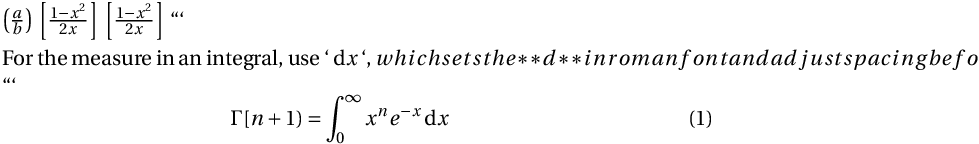# The physics Package

Documentation physics The physics package supplies a great many conveniences for typesetting physics equations. Among these is an improved version of the \left( stuff \right) structure \qty( stuff ). Works well in combination with the siunitx package. \usepackage{physics}

The following surrounds the fraction a/b with parentheses that are sized appropriately. You can also use brackets and braces.

$\qty( \frac{a}{b} )$
$\qty[ \frac{1-x^2}{2x} ]$
$\qty[ \frac{1-x^2}{2x} ]$


For the measure in an integral, use \dd{x}, which sets the d in roman font and adjust spacing before it according to context. Use \dv{x} for d/dx, \dv{f}{x} for $\df/dx$, and \dv*{f}{x} for an inline version. Similar expressions for partial derivatives use \pdv.

\begin{equation}
\Gamma[n+1) = \int_{0}^{\infty} x^n e^{-x} \dd{x}
\label{eq:gamma}
\end{equation}


For vectors, you can use \vb{F} or \vb*{\sigma) for vectors set in boldface, \va{F} or \va*{\theta} for an arrow, and \vu{a} or \vu*{\theta} for unit vectors. There is also full support for Dirac bra-ket notation.